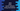# Java program to print all contents of a vector using enumeration# Java program to print vector contents :

In this tutorial, we will learn how to use enumeration to iterate over a vector in Java. Our program will first take the inputs for the vector from the user, it will then print out the inputs using enumeration.

## elements method of vector :

Vector objects have one method called elements() that can be used to get an enumeration of the components of a vector.

1. Using elements() method, we will get the enumeration of the components of the vector.
2. Then, using a while loop and hasMoreElements() method of enumeration, we will verify if any element is left in the enumeration.
3. If any element left, we will use nextElement() method to print out the value.

Let’s check the Java program first :

### Java program :

``````import java.util.Enumeration;
import java.util.Scanner;
import java.util.Vector;
public class Example {
public static void main(String[] args) {
//1
int size;
Vector<string> strVector = new Vector<>();
Scanner sc = new Scanner(System.in);
//2
System.out.println("Enter the size of the vector : ");
size = sc.nextInt();
//3
for (int i = 0; i < size; i++) {
System.out.println("Enter a string value for position " + (i + 1) + " : ");
}
//4
System.out.println("You have entered : ");
//5
Enumeration<string> enumeration = strVector.elements();
//6
while(enumeration.hasMoreElements()){
System.out.println(enumeration.nextElement());
}
}
}``````

### Explanation :

The commented numbers in the above program denote the step numbers below :

1. Create one integer variable size to store the size of the vector. Initialize one vector object strVector. This object can hold only elements of type String. Also, create one Scanner object sc to read the user inputs.
2. Ask the user to enter the size of the vector. Read the user input value and store it in size integer variable.
3. Run one for loop to take user inputs for the vector. This loop will run for ‘size’ times. On each iteration of the loop, ask the user to enter a string for the vector. Read the input using the next() method of the Scanner and add it to the vector using add() method.
4. Print out one message before printing all elements of the vector.
5. Using the elements() method, get the enumeration value from the vector. Store it in the variable ‘enumeration’.
6. Using one while loop, iterate through the enumeration. hasMoreElements() method checks if the enumeration has any more element left. If true, it moves inside the while loop and prints out the element using nextElement() method.

### Sample Output :

``````Enter the size of the vector :
3
Enter a string value for position 1 :
Hello
Enter a string value for position 2 :
World
Enter a string value for position 3 :
!!
You have entered :
Hello
World
!!

Enter the size of the vector :
5
Enter a string value for position 1 :
one
Enter a string value for position 2 :
two
Enter a string value for position 3 :
three
Enter a string value for position 4 :
four
Enter a string value for position 5 :
five
You have entered :
one
two
three
four
five``````

### Conclusion :

We have learned how to add elements to a Vector, get the list of elements as an Enumeration and how to print the elements of the Enumeration. Try to run the program and drop one comment below if you have any queries.